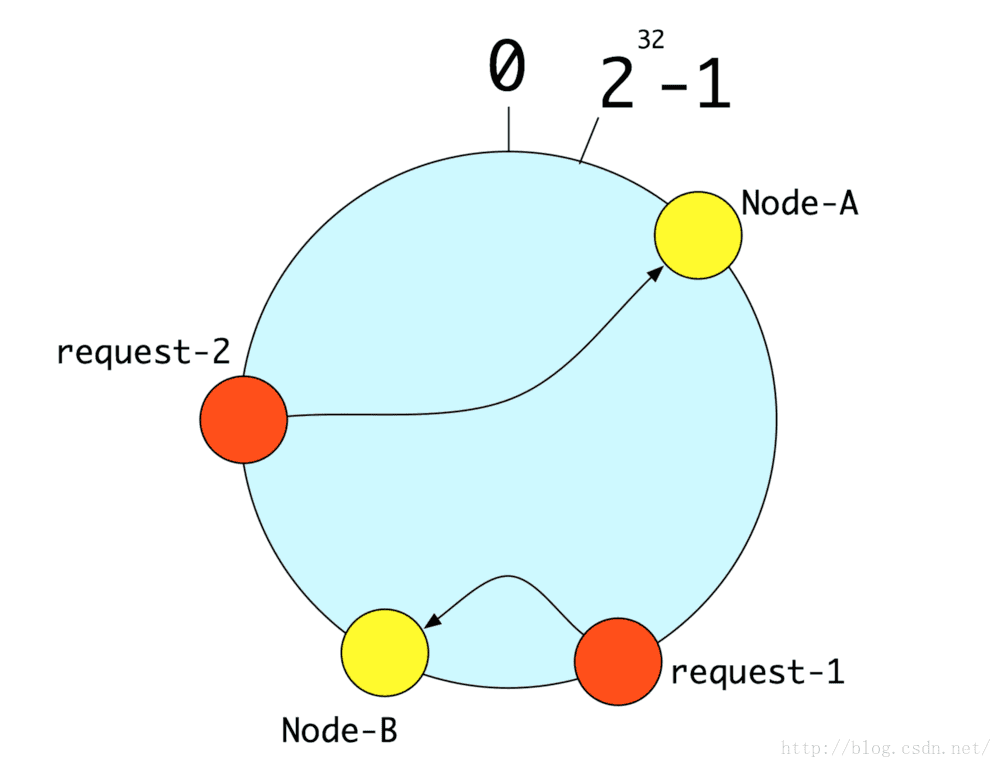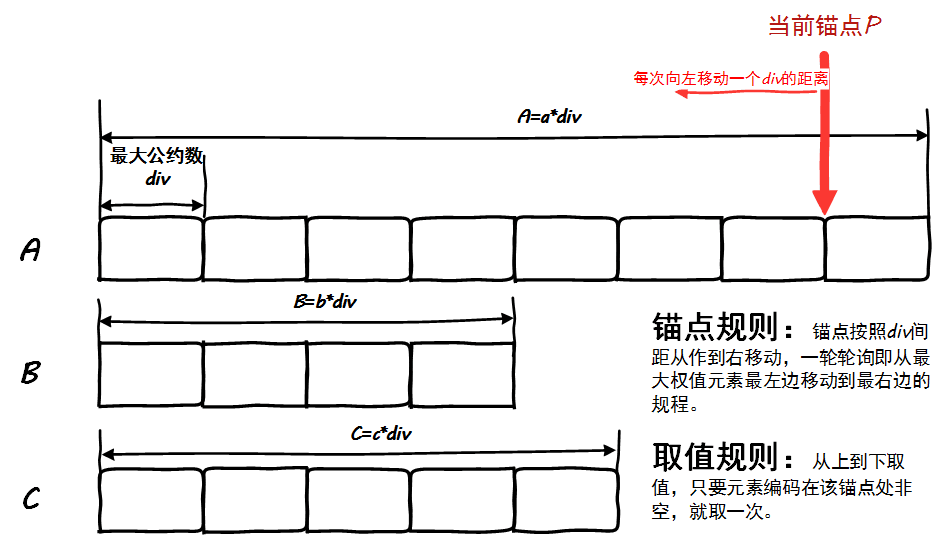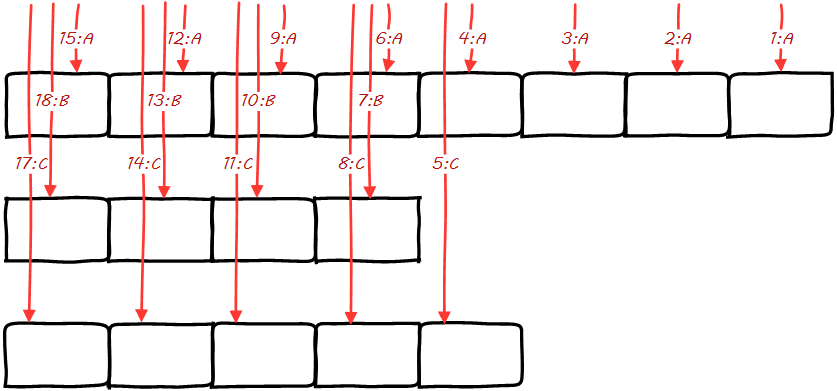• 负载均衡算法在实际中使用及其广泛，比如某个大型网站或者某个大型服务，服务器的数量可能成百上千台，这个时候是一定会有负载均衡算法的。现在总结一下常见的几种负载均衡算法。 1.轮询法(RoundRobin) 轮询法是一...
项目github地址：bitcarmanlee easy-algorithm-interview-and-practice 欢迎大家star，留言，一起学习进步
负载均衡算法在实际中使用及其广泛，比如某个大型网站或者某个大型服务，服务器的数量可能成百上千台，这个时候是一定会有负载均衡算法的。现在总结一下常见的几种负载均衡算法。
1.轮询法(RoundRobin)
轮询法是一种比较简单的算法，也比较好理解。假设后台有N台服务器，那么来请求以后，按照请求到达的先后顺序，将流量分配到服务器上，这样后端服务器上的流量都是相同的。这种方式的好处是不用关注服务器本身的负载，链接数等信息。但是坏处也比较明显，如果每台服务器的处理能力不一样，那比较强的服务器就无法发挥优势。Nginx中默认的就是轮询法。
import java.util.Arrays;
import java.util.List;

/**
* Created by WangLei on 18-6-21.
*/
public class RoundRobin {

private static List<String> servers = Arrays.asList("192.168.0.1","192.168.0.2","192.168.0.3","192.168.0.4");
private static Integer pos = 0;

public static String getServer() {
String server = null;

synchronized (pos) {
if(pos == servers.size()) {
pos = 0;
}
server = servers.get(pos);
pos++;
}

return server;
}

public static void main(String[] args) {
for(int i=0; i<10; i++) {
System.out.println(RoundRobin.getServer());
}
}
}


运行结果：
192.168.0.1
192.168.0.2
192.168.0.3
192.168.0.4
192.168.0.1
192.168.0.2
192.168.0.3
192.168.0.4
192.168.0.1
192.168.0.2

代码里面需要注意的是对于pos参数的处理，因为实际中并发的场景很常见，所以用synchronized将pos包起来，这样保证pos每次只被一个线程修改。
2.随机法
随机的方式也比较容易理解。将流量随机分配到后端某一台服务器上。根据简单的统计理论可以得知，随着流量越来越大，随机分配的实际效果越来越接近平均分配，最终的实际效果跟轮询一致。
import java.util.Arrays;
import java.util.List;
import java.util.Random;

/**
* Created by WangLei on 18-6-21.
*/
public class RandomMethod {

private static List<String> servers = Arrays.asList("192.168.0.1","192.168.0.2","192.168.0.3","192.168.0.4");
private static int pos = 0;

public static String getServer() {
String server = null;

Random random = new Random();
int randomPos = random.nextInt(servers.size());

server = servers.get(randomPos);

return server;
}

public static void main(String[] args) {
for(int i=0; i<10; i++) {
System.out.println(RandomMethod.getServer());
}
}

}

192.168.0.4
192.168.0.4
192.168.0.1
192.168.0.4
192.168.0.3
192.168.0.1
192.168.0.4
192.168.0.3
192.168.0.1
192.168.0.3

根据代码可以看出，在并发的情况下，随机方式不需要像轮询一样加锁，并发能力比轮询要强。
3.主备算法
这个算法核心的思想是将请求尽量的放到某个固定机器的服务上，而其他机器的服务则用来做备份，如果出现问题就切换到另外的某台机器的服务上。这么做的好处之一就是每个流量分配到哪个服务器上是固定的，在某些场合会比较方便。
比如我们下面实现一个简单的主备，根据客户端的ip将流量分配到固定的机器上。
import java.util.Arrays;
import java.util.List;

/**
* Created by WangLei on 18-6-21.
*/
public class HashMethod {

private static List<String> servers = Arrays.asList("192.168.0.1","192.168.0.2","192.168.0.3","192.168.0.4");
private static int pos = 0;

public static String getServer(String ip) {
String server = null;

int hashCode = ip.hashCode();
pos = hashCode % servers.size();

server = servers.get(pos);

return server;
}

public static void main(String[] args) {
String ip = "192.168.255.254";
System.out.println(HashMethod.getServer(ip));
}
}

192.168.0.3

4.一致性Hash
一致性Hash算法通过一个叫做一致性Hash环的数据结构实现Key到后端服务器的Hash映射。如果是缓存服务，实现的则是Key到缓存服务器的Hash映射。从网上找了一张示意图。算法的具体逻辑如下：将[0,2^32)所有的整数投射到一个圆上，然后再将你的机器的唯一编码（比如：IP）通过hash运算得到的整数也投射到这个圆上（Node-A、Node-B）。如果一个请求来了，就将这个请求的唯一编码（比如：用户id）通过hash算法运算得到的整数也投射到这个圆上（request-1、request-2），通过顺时针方向，找到第一个对应的机器。
一致性Hash需要解决的是以下两个问题： 1、散列的不变性：就是同一个请求（比如：同一个用户id）尽量的落入到一台机器，不要因为时间等其他原因，落入到不同的机器上了； 2、异常以后的分散性：当某些机器坏掉（或者增加机器），原来落到同一台机器的请求（比如：用户id为1，101，201），尽量分散到其他机器，不要都落入其他某一台机器。这样对于系统的冲击和影响最小。 一致Hash算法用的最多的场景，就是分配cache服务。将某一个用户的数据缓存在固定的某台服务器上，那么我们基本上就不用多台机器都缓存同样的数据，这样对我们提高缓存利用率有极大的帮助。 (此部分来自https://blog.csdn.net/zgwangbo/article/details/51533657）
先看一个一致性Hash的简单实现版本。
import java.util.Arrays;
import java.util.List;
import java.util.SortedMap;
import java.util.TreeMap;

/**
* Created by WangLei on 18-6-21.
*/
public class ConsisentHashNoVirtualNode {

private static String[] serversarray = {"192.168.0.0:111", "192.168.0.1:111", "192.168.0.2:111","192.168.0.3:111", "192.168.0.4:111"};
private static List<String> servers = Arrays.asList(serversarray);

private static SortedMap<Integer, String> sortedMap = new TreeMap<>();

static {
for(int i=0; i<servers.size(); i++) {
int hash = hashCode(servers.get(i));
System.out.println(servers.get(i) + " join collections, hashcode is: " + hash);
sortedMap.put(hash, servers.get(i));
}
System.out.println();
}

// 重写hashcode方法，使用FNV1_32_HASH算法，让节点在hash环上分布更均匀。
private static int hashCode(String ip) {
final int p = 16777619;
int hash = (int)2166136261L;
for(int i=0; i<ip.length(); i++) {
hash = (hash ^ ip.charAt(i)) * p;
}
hash += hash << 13;
hash ^= hash >> 7;
hash += hash << 3;
hash ^= hash >> 17;
hash += hash << 5;

if(hash < 0) {
hash = Math.abs(hash);
}

return hash;
}

private static String getServer(String client) {
int hash = hashCode(client);
// 得到大于该Hash值的所有Map。注意有可能subMap一个值没有，此时默认分给第一个server。
SortedMap<Integer, String> subMap = sortedMap.tailMap(hash);
if(subMap != null && subMap.size() > 0) {
int i = subMap.firstKey();
return subMap.get(i);
} else {
return sortedMap.get(hashCode(servers.get(0)));
}
}

public static void main(String[] args) {
String[] clients =  {"127.0.0.1:22", "221.226.0.1:80", "10.211.0.1:8080"};
//String[] clients =  {"127.0.0.1:22", "221.226.0.1:80"};

for(int i=0; i<clients.length; i++) {
System.out.println(clients[i] + " hashcode is: " + hashCode(clients[i]) + ", the router node is: " + getServer(clients[i]));
}
}
}

运行结果：
192.168.0.0:111 join collections, hashcode is: 575774686
192.168.0.1:111 join collections, hashcode is: 8518713
192.168.0.2:111 join collections, hashcode is: 1361847097
192.168.0.3:111 join collections, hashcode is: 1171828661
192.168.0.4:111 join collections, hashcode is: 1764547046

127.0.0.1:22 hashcode is: 1739501660, the router node is: 192.168.0.4:111
221.226.0.1:80 hashcode is: 99109700, the router node is: 192.168.0.0:111
10.211.0.1:8080 hashcode is: 1976495495, the router node is: 192.168.0.0:111

上面的一致性Hash算法实现，可以在很大程度上解决很多分布式环境下不好的路由算法导致系统伸缩性差的问题，但是会带来另外一个问题：负载不均。
比如说有Hash环上有A、B、C三个服务器节点，分别有100个请求会被路由到相应服务器上。现在在A与B之间增加了一个节点D，这导致了原来会路由到B上的部分节点被路由到了D上，这样A、C上被路由到的请求明显多于B、D上的，原来三个服务器节点上均衡的负载被打破了。某种程度上来说，这失去了负载均衡的意义，因为负载均衡的目的本身就是为了使得目标服务器均分所有的请求。
解决这个问题的办法是引入虚拟节点，其工作原理是：将一个物理节点拆分为多个虚拟节点，并且同一个物理节点的虚拟节点尽量均匀分布在Hash环上。采取这样的方式，就可以有效地解决增加或减少节点时候的负载不均衡的问题。
import java.util.LinkedList;
import java.util.List;
import java.util.SortedMap;
import java.util.TreeMap;

/**
* Created by WangLei on 18-6-21.
*/
public class ConsisenthashWithVituralNode {

private static String[] serversarray = {"192.168.0.1","192.168.0.2","192.168.0.3","192.168.0.4"};

private static List<String> realservers = new LinkedList<>();

private static final int VIRTUAL_NUM = 5;

private static SortedMap<Integer, String> sortedMap = new TreeMap<>();

static {
for(int i=0; i<serversarray.length; i++) {
}
//双层循环，每个实际节点都添加对应的虚拟节点
for(String ip : realservers) {
for(int i = 0; i < VIRTUAL_NUM; i++) {
String virtualNode = ip + "#" + String.valueOf(i);
int hash = hashCode(virtualNode);
System.out.println("virtual node of " + virtualNode + " add, hash is: " + hash);
sortedMap.put(hash, virtualNode);
}
}
System.out.println();
}

private static int hashCode(String input) {
final int p = 16777619;
int hash = (int)2166136261L;
for(int i=0; i<input.length(); i++) {
hash = (hash ^ input.charAt(i)) * p;
}
hash += hash << 13;
hash ^= hash >> 7;
hash += hash << 3;
hash ^= hash >> 17;
hash += hash << 5;

if(hash < 0) {
hash = Math.abs(hash);
}

return hash;
}

private static String getServer(String client) {
String virtualNode = null;
int hash = hashCode(client);

SortedMap<Integer, String> subMap = sortedMap.tailMap(hash);
if(subMap != null && subMap.size() > 0) {
int i = subMap.firstKey();
virtualNode = subMap.get(i);
} else {
virtualNode = sortedMap.get(serversarray);
}
return virtualNode.substring(0, virtualNode.indexOf("#"));
}

public static void main(String[] args) {
String[] clients =  {"127.0.0.1:22", "110.226.0.1:80", "255.21.0.1:8080"};
for(int i=0; i<clients.length; i++) {
System.out.println(clients[i] + " hashcode is: " + hashCode(clients[i]) + " the router node is: " + getServer(clients[i]));
}
}
}

运行结果：
virtual node of 192.168.0.1#0 add, hash is: 1267794962
virtual node of 192.168.0.1#1 add, hash is: 1405473402
virtual node of 192.168.0.1#2 add, hash is: 520282580
virtual node of 192.168.0.1#3 add, hash is: 902681916
virtual node of 192.168.0.1#4 add, hash is: 705135863
virtual node of 192.168.0.2#0 add, hash is: 938864723
virtual node of 192.168.0.2#1 add, hash is: 697737174
virtual node of 192.168.0.2#2 add, hash is: 758522939
virtual node of 192.168.0.2#3 add, hash is: 1305037326
virtual node of 192.168.0.2#4 add, hash is: 1002536378
virtual node of 192.168.0.3#0 add, hash is: 392944983
virtual node of 192.168.0.3#1 add, hash is: 2046386183
virtual node of 192.168.0.3#2 add, hash is: 1604877649
virtual node of 192.168.0.3#3 add, hash is: 95135893
virtual node of 192.168.0.3#4 add, hash is: 2030051947
virtual node of 192.168.0.4#0 add, hash is: 1614569944
virtual node of 192.168.0.4#1 add, hash is: 744068005
virtual node of 192.168.0.4#2 add, hash is: 1628537157
virtual node of 192.168.0.4#3 add, hash is: 537291676
virtual node of 192.168.0.4#4 add, hash is: 1173079769

127.0.0.1:22 hashcode is: 1739501660 the router node is: 192.168.0.3
110.226.0.1:80 hashcode is: 1320900860 the router node is: 192.168.0.1
255.21.0.1:8080 hashcode is: 1187650066 the router node is: 192.168.0.1

展开全文一致性哈希 轮询
• F5负载均衡算法详解:静态负载均衡算法和动态负载均衡算法
• 首先给大家说下 upstream 这个配置的，这个配置是写一组被代理的服务器地址，然后配置负载均衡算法. upstream testapp { server 10.0.105.199:8081; server 10.0.105.202:8081; } server { .... location / ...
upstream配置
首先给大家说下 upstream 这个配置的，这个配置是写一组被代理的服务器地址，然后配置负载均衡的算法.
upstream testapp {
server 10.0.105.199:8081;
server 10.0.105.202:8081;
}
server {
....
location / {
proxy_pass  http://testapp;  #请求转向 testapp 定义的服务器列表
}

1、负载均衡算法
upstream 支持4种负载均衡调度算法
A、轮询(默认):每个请求按时间顺序逐一分配到不同的后端服务器;
B、ip_hash:每个请求按访问IP的hash结果分配，同一个IP客户端固定访问一个后端服务器。可以保证来自同一ip的请求被打到固定的机器上，可以解决session问题。
C、url_hash:按访问url的hash结果来分配请求，使每个url定向到同一个后端服务器。
D、fair:这是比上面两个更加智能的负载均衡算法。此种算法可以依据页面大小和加载时间长短智能地进行负载均衡，也就是根据后端服务器的响应时间来分配请求，响应时间短的优先分配。Nginx本身是不支持 fair的，如果需要使用这种调度算法，必须下载Nginx的 upstream_fair模块。
2、配置实例
1、热备：如果你有2台服务器，当一台服务器发生事故时，才启用第二台服务器给提供服务。服务器处理请求的顺序：AAAAAA突然A挂啦，BBBBBBBBBBBBBB…
upstream myweb {
server 172.17.14.2:8080;
server 172.17.14.3:8080 backup;  #热备
}

2、轮询：nginx默认就是轮询其权重都默认为1，服务器处理请求的顺序：ABABABABAB…
upstream myweb {
server 172.17.14.2:8080;
server 172.17.14.3:8080;
}

3、加权轮询：跟据配置的权重的大小而分发给不同服务器不同数量的请求。如果不设置，则默认为1。下面服务器的请求顺序为：ABBABBABBABBABB…
upstream myweb {
server 172.17.14.2:8080 weight=1;
server 172.17.14.3:8080 weight=2;
}

4、ip_hash:nginx会让相同的客户端ip请求相同的服务器。
upstream myweb {
server 172.17.14.2:8080;
server 172.17.14.3:8080;
ip_hash;
}

5、nginx负载均衡配置状态参数
down，表示当前的server暂时不参与负载均衡。backup，预留的备份机器。当其他所有的非backup机器出现故障或者忙的时候，才会请求backup机器，因此这台机器的压力最轻。max_fails，允许请求失败的次数，默认为1。当超过最大次数时，返回proxy_next_upstream 模块定义的错误。fail_timeout，在经历了max_fails次失败后，暂停服务的时间单位秒。max_fails可以和fail_timeout一起使用。
 upstream myweb {
server 172.17.14.2:8080 weight=2 max_fails=2 fail_timeout=2;
server 172.17.14.3:8080 weight=1 max_fails=2 fail_timeout=1;
#max_fails=2 fail_timeout=1
#用于判断后端节点状态，所用到两个参数。
#Nginx基于连接探测，如果发现后端异常，在单位周期为fail_timeout设置的时间，
#中达到max_fails次数，这个周期次数内，如果后端同一个节点不可用，那么接将把节点标记为不可用，
#并等待下一个周期（同样时常为fail_timeout）再一次去请求，判断是否连接是否成功。
}

如果你像跟多更深入的了解 nginx 的负载均衡算法，nginx官方提供一些插件大家可以了解下。
ip_hash
ip_hash使用源地址哈希算法，将同一客户端的请求总是发往同一个后端服务器，除非该服务器不可用。
ip_hash语法：
upstream backend {
ip_hash;
server backend1.example.com;
server backend2.example.com;
server backend3.example.com down;
}

ip_hash简单易用，但有如下问题： 当后端服务器宕机后，session会丢失； 来自同一局域网的客户端会被转发到同一个后端服务器，可能导致负载失衡；
sticky模块
语法：
upstream backend {
server backend1.example.com;
server backend2.example.com;
}

使用后端服务器自身通过相关机制保持session同步，如：使用数据库、redis、memcached 等做session复制
展开全文nginx 服务器
• 目前haproxy支持的负载均衡算法有如下8种： 1、roundrobin 表示简单的轮询，每个服务器根据权重轮流使用，在服务器的处理时间平均分配的情况下这是最流畅和公平的算法。该算法是动态的，对于实例启动慢的服务器...

目前haproxy支持的负载均衡算法有如下8种：
1、roundrobin
表示简单的轮询，每个服务器根据权重轮流使用，在服务器的处理时间平均分配的情况下这是最流畅和公平的算法。该算法是动态的，对于实例启动慢的服务器权重会在运行中调整。最大支持4095个后端主机；
2、leastconn
连接数最少的服务器优先接收连接。leastconn建议用于长会话服务，例如LDAP、SQL、TSE等，而不适合短会话协议。如HTTP.该算法是动态的，对于实例启动慢的服务器权重会在运行中调整。
3、static-rr
每个服务器根据权重轮流使用，类似roundrobin，但它是静态的，意味着运行时修改权限是无效的。另外，它对服务器的数量没有限制。
该算法一般不用；
4、source
对请求源IP地址进行哈希，用可用服务器的权重总数除以哈希值，根据结果进行分配。只要服务器正常，同一个客户端IP地址总是访问同一个服务器。如果哈希的结果随可用服务器数量而变化，那么客户端会定向到不同的服务器；
该算法默认是静态的，所以运行时修改服务器的权重是无效的，但是算法会根据“hash-type”的变化做调整。
5、uri
表示根据请求的URI左端（问号之前）进行哈希，用可用服务器的权重总数除以哈希值，根据结果进行分配。只要服务器正常，同一个URI地址总是访问同一个服务器。一般用于代理缓存和反病毒代理，以最大限度的提高缓存的命中率。该算法只能用于HTTP后端；
该算法一般用于后端是缓存服务器；
该算法默认是静态的，所以运行时修改服务器的权重是无效的，但是算法会根据“hash-type”的变化做调整。
6、url_param
在HTTP GET请求的查询串中查找<param>中指定的URL参数，基本上可以锁定使用特制的URL到特定的负载均衡器节点的要求；
该算法一般用于将同一个用户的信息发送到同一个后端服务器；
该算法默认是静态的，所以运行时修改服务器的权重是无效的，但是算法会根据“hash-type”的变化做调整。
7、hdr(name)
在每个HTTP请求中查找HTTP头<name>，HTTP头<name>将被看作在每个HTTP请求，并针对特定的节点；
如果缺少头或者头没有任何值，则用roundrobin代替；
该算法默认是静态的，所以运行时修改服务器的权重是无效的，但是算法会根据“hash-type”的变化做调整。
该算法默认是静态的，所以运行时修改服务器的权重是无效的，但是算法会根据“hash-type”的变化做调整。
转自：https://my.oschina.net/BambooLi/blog/506397

转载于:https://www.cnblogs.com/wensiyang0916/p/6490405.html
展开全文• 应用交换技术的负载均衡算法 应用交换技术里主要包括四项关键的技术： 截获和检查流量 服务器监控健康检查 负载均衡算法 会话保持 截获和检查流量保证只有合适的数据包才能通过； 服务器监控和健康检查随时了解...
• ## IPVS和Nginx两种WRR负载均衡算法详解

千次阅读 热门讨论 2018-04-28 05:23:40
忆起与人交流一个负载均衡问题时，偶然聊到了WRR算法，就必然要记下些什么，以表示曾经聊过这个话题，作此文以记之！ 简介 在负载均衡场景中，我们经常需要对一组服务器做加权轮询均衡调用，即适配一个...
动机
五一临近，四月也接近尾声，五一节乃小长假的最后一天。今天是最后一天工作日，竟然感冒了，半夜里翻来覆去无法安睡，加上窗外大飞机屋里小飞机(也就是蚊子)的骚扰，实在是必须起来做点有意义的事了！
忆起与人交流一个负载均衡问题时，偶然聊到了WRR算法，就必然要记下些什么，以表示曾经聊过这个话题，作此文以记之！
简介
在负载均衡场景中，我们经常需要对一组服务器做加权轮询均衡调用，即适配一个叫做WRR(Weighted Round-Robin Scheduling)的算法。本文的主要内容就是分析常见的两种WRR算法，即Linux IPVS的WRR算法和Nginx的WRR算法，并试图做出二者的比较。
当然了，负载均衡的算法非常多，但很难在一篇技术随笔中盖以全貌，与其说不透，不如干脆不说，因此本文的内容仅仅包含两种常见的WRR算法。
Linux内核IPVS使用的WRR算法
这里接不介绍IPVS了，直接切入算法本身，详见net/netfilter/ipvs/ip_vs_wrr.c中的结构体：
static struct ip_vs_scheduler ip_vs_wrr_scheduler = {
.name =         "wrr",
.refcnt =       ATOMIC_INIT(0),
.module =       THIS_MODULE,
.init_service =     ip_vs_wrr_init_svc,
.done_service =     ip_vs_wrr_done_svc,
.del_dest =     ip_vs_wrr_dest_changed,
.upd_dest =     ip_vs_wrr_dest_changed,
.schedule =     ip_vs_wrr_schedule,
};
这里重点关注schedule 回调函数ip_vs_wrr_schedule。
为了让事情更加直观，不至于陷入到Linux内核源码IPVS复杂业务逻辑的深渊，这里给出其Wiki上上的写法，摘自：http://kb.linuxvirtualserver.org/wiki/Weighted_Round-Robin_Scheduling：
Supposing that there is a server set S = {S0, S1, …, Sn-1};
W(Si) indicates the weight of Si;
i indicates the server selected last time, and i is initialized with -1;
cw is the current weight in scheduling, and cw is initialized with zero;
max(S) is the maximum weight of all the servers in S;
gcd(S) is the greatest common divisor of all server weights in S;

while (true) {
i = (i + 1) mod n;
if (i == 0) {
cw = cw - gcd(S);
if (cw <= 0) {
cw = max(S);
if (cw == 0)
return NULL;
}
}
if (W(Si) >= cw)
return Si;
}
如果你还是没有一个直观上的感受，下面是我写的一个简单的能run的程序，直接编译运行即可：
#include <stdio.h>
#include <stdlib.h>

struct entry {
int weight;
};

struct entry *g_entry = NULL;
int max_weight = 0;
int curr_weight = 0;
int divisor = 0;
int iter = -1;

int gcd(int a, int b)
{
if (a == 0) {
return b;
}
return gcd(b%a, a);
}

struct entry *next(int size)
{

struct entry *ent;
while (1) {
iter = (iter + 1) % size;
if (iter == 0) {
curr_weight = curr_weight - divisor;
if (curr_weight <= 0) {
curr_weight = max_weight;
}
}
ent = &g_entry[iter];
if (ent->weight >= curr_weight) {
return ent;
}
}
}

int main(int argc, char **argv)
{
int size = atoi(argv);
int i = 0;
int total = 0;

g_entry = (struct entry *)calloc(size, sizeof(struct entry));
for (i = 0; i < size; i++) {
struct entry *ent = &g_entry[i];
ent->weight = atoi(argv[i+2]);
total += ent->weight;
if (ent->weight > max_weight) {
max_weight = ent->weight;
}
divisor = gcd(divisor, ent->weight);
}

for (i = 0; i < total; i++) {
struct entry *ent = next(size);
printf("[LAST]: %d\n", ent->weight);
}

}
你可以这样使用这个程序：
# 这里生成一个3(第一个参数)个元素的集合，其权值分别为5,1,1(后面的参数)
./a.out 3 5 1 1
简单的证明和分析
这个算法给出的结果总是正确的吗？回答这个问题我觉得非常简单直观，请看下图：按照上图中的规则，取元素的顺序则是：在数学上证明算法的正确性似乎也不难，设一个元素的权值为

Wi

W

i

$W_i$，那么它在锚点一轮轮询中被选中的次数就是

Widiv

W

i

d

i

v

$\dfrac{W_i}{div}$，可见，对于一个固定的序列，其

div

d

i

v

$div$一定，一个元素被选中的次数与其权值成正比，这便符合了算法的要求。
问题
观察上面的图，如果一个集合中最大权值的元素和次大权值的元素相隔太远，那么这个算法在选元素的时候是不会把权值大的元素打散的，比如：
root@debian:/home/zhaoya# ./a.out 2 5 1
[LAST]: 5
[LAST]: 5
[LAST]: 5
[LAST]: 5
[LAST]: 5
[LAST]: 1
映射回负载均衡的真实场景，这显然会对某些大权值的服务器造成很大的压力，因此对这个算法的改进或者说换另外一个算法是一件必须要做的事。接下来我们就开始分析一个结果序列更加平均的WRR算法，即Nginx服务器中使用的WRR算法。

Nginx使用的WRR算法
关于这个算法，具体的描述详见：  https://github.com/phusion/nginx/commit/27e94984486058d73157038f7950a0a36ecc6e35
和分析IPVS之WRR算法的做法一样，我依然给出一个能run的代码，其运行方法与上述IPVS的算法完全一致：
#include <stdio.h>
#include <stdlib.h>

struct entry {
int weight;
int curr_weight;
};

struct entry *curr_entry = NULL;
struct entry *g_entry = NULL;

struct entry *next(struct entry *entrys, int size)
{

struct entry *ent;
int i = 0, total = 0;
for (i = 0; i < size; i++) {
ent = &entrys[i];
ent->curr_weight += ent->weight;
total += ent->weight;
if (curr_entry == NULL || ent->curr_weight > curr_entry->curr_weight) {
curr_entry = ent;
}
}
curr_entry->curr_weight -= total;
for (i = 0; i < size; i++) {
ent = &entrys[i];
}
return curr_entry;
}

int main(int argc, char **argv)
{
int size = atoi(argv);
int i = 0;
int total = 0;

g_entry = (struct entry *)calloc(size, sizeof(struct entry));
for (i = 0; i < size; i++) {
struct entry *ent = &g_entry[i];
ent->weight = atoi(argv[i+2]);
total += ent->weight;
}

for (i = 0; i < total; i++) {
struct entry *ent = next(g_entry, size);
printf("[LAST]: %d\n", ent->weight);
}

}
以上就是Nginx所采用的WRR算法的代码描述，在大多数情况下，采用这种算法是一个不错的选择。即满足了固定的加权平均，又使得元素的选择尽可能地分散开来，非常精妙！
该算法与IPVS的WRR按照某种规则和组织静态遍历完全不同，它完全是一个动态的过程，因此除非用动画，否则一张图无法展示全貌。我用简单的3个元素加权来描述一下这个算法。假设有3个具有不同权值的

{A:a,B:b,C:c}

{

A

:

a

,

B

:

b

,

C

:

c

}

$\{A:a,B:b,C:c\}$元素供选择，在一轮轮询中，3个元素分别被递加其权值的递增量为：
元素

A

A

$A$递增a$a$$a$的量：

a×(a+b+c)

a

×

(

a

+

b

+

c

)

$a\times (a+b+c)$  元素

B

B

$B$递增b$b$$b$选中的量：

b×(a+b+c)

b

×

(

a

+

b

+

c

)

$b\times (a+b+c)$  元素

C

C

$C$递增c$c$$c$选中的量：

c×(a+b+c)

c

×

(

a

+

b

+

c

)

$c\times (a+b+c)$
每选中一个元素，将会从其递增量中减去

(a+b+c)

(

a

+

b

+

c

)

$(a+b+c)$，那么很显然，问题部分地被转化为求出每个元素递增量中包含多少个递减量便是该元素会被选中的次数。最终，我们承认下面的解是一个正确的解：
元素

A

A

$A$被选中的次数：a×(a+b+c)a+b+c$\frac{a×\left(a+b+c\right)}{a+b+c}$$\dfrac {a\times (a+b+c)}{a+b+c}$次  元素

B

B

$B$被选中的次数：b×(a+b+c)a+b+c$\frac{b×\left(a+b+c\right)}{a+b+c}$$\dfrac {b\times (a+b+c)}{a+b+c}$次  元素

C

C

$C$被选中的次数：c×(a+b+c)a+b+c$\frac{c×\left(a+b+c\right)}{a+b+c}$$\dfrac {c\times (a+b+c)}{a+b+c}$次
然而，这个解是唯一的解吗？如何证明这是唯一解？这便是一个数学问题。理解并使用该算法是完全没有问题的，coders深谙此道，然而想要彻底理解它，则必须要证明在算法的操作下，最终得到的解是唯一解，接下来我就简单用反证法来证明一下。
算法的描述
这个算法很有意思，所有集合一开始各就各位初始化自己的

Wcurri

W

c

u

r

r

i

$W_{curr_i}$为自己的权值，然后谁获胜(即其

Wcurri

W

c

u

r

r

i

$W_{curr_i}$最大)，谁就要减掉所有元素的权值之和

(a+b+c)

(

a

+

b

+

c

)

$(a+b+c)$，接下来继续推进一步，每一个元素再加上自己的权值…最终我们发现，每一步推进过程，每一个元素各自增加自身的权值，然后获胜者把所有元素增加的权值一次性做减法，这意味着，任何时候，在选择元素前：

ΣWcurri=a+b+c

Σ

W

c

u

r

r

i

=

a

+

b

+

c

$\Sigma W_{curr_i} = a+b+c$
而选择了

Wcurri

W

c

u

r

r

i

$W_{curr_i}$最大的元素后：

ΣWcurri=0

Σ

W

c

u

r

r

i

=

0

$\Sigma W_{curr_i} = 0$
这像不像古代军队弓箭手放乱箭的过程，简直太像了！同时，这是一种非积累即时消费的模型，即获胜者一次性消费掉其它选手在本轮中获取的配额。这种非积累特性抹掉了很多潜在的记忆阻止了幂律产生作用，让结果散列地更均匀。

算法正确性证明
假设在集合

{A:a,B:b,C:c}

{

A

:

a

,

B

:

b

,

C

:

c

}

$\{A:a,B:b,C:c\}$尚未取够

(a+b+c)

(

a

+

b

+

c

)

$(a+b+c)$个元素的时候，权重为

a

a

$a$的元素A$A$$A$已经取满了

a

a

$a$个，此时：

Wcurra=N×a−(a−1)(a+b+c)${W}_{cur{r}_{a}}=N×a-\left(a-1\right)\left(a+b+c\right)$$W_{curr_a}=N\times a - (a-1)(a+b+c)$

Wcurrb=N×b−mb1(a+b+c)

W

c

u

r

r

b

=

N

×

b

−

m

b

1

(

a

+

b

+

c

)

$W_{curr_b}=N\times b - m_{b_1}(a+b+c)$

Wcurrc=N×c−mc1(a+b+c)

W

c

u

r

r

c

=

N

×

c

−

m

c

1

(

a

+

b

+

c

)

$W_{curr_c}=N\times c - m_{c_1}(a+b+c)$
由算法基本逻辑，我们知道上面

3

3

$3$式子相加等于(a+b+c)$\left(a+b+c\right)$$(a+b+c)$：

ΣWcurri=N×(a+b+c)−(a−1+mb1+mc1)(a+b+c)=(a+b+c)

Σ

W

c

u

r

r

i

=

N

×

(

a

+

b

+

c

)

−

(

a

−

1

+

m

b

1

+

m

c

1

)

(

a

+

b

+

c

)

=

(

a

+

b

+

c

)

$\Sigma W_{curr_i} = N\times (a+b+c)-(a-1+m_{b_1}+m_{c_1})(a+b+c)=(a+b+c)$
化简得到：

N=a+mb1+mc1

N

=

a

+

m

b

1

+

m

c

1

$N=a+m_{b_1}+m_{c_1}$

(1)

(

1

)

$(1)$
现在假设，在取到第

T

T

$T$(N<T<a+b+c)$\left(N$(N个的时候，又取了一个权重为

a

a

$a$的元素A$A$$A$，则此时根据算法逻辑计算

Wcurri

W

c

u

r

r

i

$W_{curr_i}$：

Wcurra=T×a−(a+mT−1)(a+b+c)

W

c

u

r

r

a

=

T

×

a

−

(

a

+

m

T

−

1

)

(

a

+

b

+

c

)

$W_{curr_a}=T\times a - (a+m_T-1)(a+b+c)$

mT

m

T

$m_T$为此间取到

A

A

$A$的次数
Wcurrb=T×b−mb2(a+b+c)${W}_{cur{r}_{b}}=T×b-{m}_{{b}_{2}}\left(a+b+c\right)$$W_{curr_b}=T\times b - m_{b_2}(a+b+c)$

Wcurrc=T×c−mc2(a+b+c)

W

c

u

r

r

c

=

T

×

c

−

m

c

2

(

a

+

b

+

c

)

$W_{curr_c}=T\times c - m_{c_2}(a+b+c)$
又因为取到权值为

a

a

$a$的元素A$A$$A$的条件是此时其

Wcurr

W

c

u

r

r

$W_curr$最大，则有：

T×a−(a+mT−1)(a+b+c)>T×b−mb2(a+b+c)

T

×

a

−

(

a

+

m

T

−

1

)

(

a

+

b

+

c

)

>

T

×

b

−

m

b

2

(

a

+

b

+

c

)

$T\times a - (a+m_T-1)(a+b+c) > T\times b - m_{b_2}(a+b+c)$

T×a−(a+mT−1)(a+b+c)>T×c−mc2(a+b+c)

T

×

a

−

(

a

+

m

T

−

1

)

(

a

+

b

+

c

)

>

T

×

c

−

m

c

2

(

a

+

b

+

c

)

$T\times a - (a+m_T-1)(a+b+c) > T\times c - m_{c_2}(a+b+c)$
化简上面式子：

T×(a−b)>(a+mT−1−mb2)(a+b+c)

T

×

(

a

−

b

)

>

(

a

+

m

T

−

1

−

m

b

2

)

(

a

+

b

+

c

)

$T\times (a-b) >(a+m_T-1-m_{b_2})(a+b+c)$

T×(a−c)>(a+mT−1−mc2)(a+b+c)

T

×

(

a

−

c

)

>

(

a

+

m

T

−

1

−

m

c

2

)

(

a

+

b

+

c

)

$T\times (a-c) >(a+m_T-1-m_{c_2})(a+b+c)$
根据条件

N<T<a+b+c

N

<

T

<

a

+

b

+

c

$N代入，则有：

T×(a−b)>(a+mT−1−mb2)×T

T

×

(

a

−

b

)

>

(

a

+

m

T

−

1

−

m

b

2

)

×

T

$T\times (a-b) > (a+m_T-1-m_{b_2})\times T$

T×(a−c)>(a+mT−1−mc2)×T

T

×

(

a

−

c

)

>

(

a

+

m

T

−

1

−

m

c

2

)

×

T

$T\times (a-c) > (a+m_T-1-m_{c_2})\times T$
最终，我们得到两个不等式：

mb2−b>mT−1

m

b

2

−

b

>

m

T

−

1

$m_{b_2}-b > m_T-1$

mc2−c>mT−1

m

c

2

−

c

>

m

T

−

1

$m_{c_2}-c > m_T-1$
由于我们是在第

T

T

$T$次取的第a+1$a+1$$a+1$个权值为

a

a

$a$的元素A$A$$A$，所以这里的

m

m

$m$等于1$1$$1$，则有：

mb2−b>0

m

b

2

−

b

>

0

$m_{b_2}-b > 0$

mc2−c>0

m

c

2

−

c

>

0

$m_{c_2}-c > 0$
现在谜底要揭开了！由于我们假设在集合

{A:a,B:b,C:c}

{

A

:

a

,

B

:

b

,

C

:

c

}

$\{A:a,B:b,C:c\}$取完

a+b+c

a

+

b

+

c

$a+b+c$个元素之后，权值为

a

a

$a$的元素A$A$$A$的取量多于

a

a

$a$个，那么权值为b$b$$b$和

c

c

$c$的元素B$B$$B$和

C

C

$C$取量必然至少有一个要少于自己权重数值，而上面的不等式表明，若需要满足权值为a$a$$a$的元素

A

A

$A$的取量多于a$a$$a$个，权值为

b

b

$b$和c$c$$c$的元素

B

B

$B$和C$C$$C$取量也必须同时多于它们的权值！这显然最终会造成一个矛盾：

ma+mb+mc>a+b+c

m

a

+

m

b

+

m

c

>

a

+

b

+

c

$m_a+m_b+m_c > a+ b+c$
因此，假设是错误的！即：
权值为

a

a

$a$的元素A$A$$A$在一轮轮询之后的取量不可能多于

a

a

$a$个

那么，能不能少于a$a$$a$个呢？这个问题很简单！既然

A

A

$A$元素少于a$a$$a$个，那么在总量

a+b+c

a

+

b

+

c

$a+b+c$一定的情况下，一定有别的元素的取量多于自己的权值，因此问题还是会转化为上面我们反证的问题，这实际上是同一个问题！

好了，本节我们证明了Nginx里面的这个WRR算法是正确的，即通过算法指示的操作，算法轮询结束后，会严格按照元素的权值比例分配被轮询的次数。 为什么比IPVS的WRR要好？ 这个问题其实很难回答，因为很难有一个确定的标准。我咨询过很多大师大神，得到的答案几乎都是从概率，global state的变更频率以及最大熵的角度来分析，然而这些对于回到这个问题有点复杂了。因为我知道Nginx的WRR算法也远不是最好的，其序列分布也不满足最大熵…   所以，我把问题化简，我只要能求出一个权值最大的元素在序列一开始时连续分布的最大上界就基本OK了，如果我求出的这个上界小于其权值

Wi

W

i

$W_i$，那就可以说明不可能所有的最大权值的元素完全连续分布，但确实要连续分布一点点，这便打散了整个分布，这已经比IPVS的WRR算法最终的序列分布要好了。   现在让我们开始。
假设元素

A

A

$A$的权值最大，为a$a$$a$，设其连续分布

x

x

$x$次，则有：

x×a−(x−1)(a+b+c)>0$x×a-\left(x-1\right)\left(a+b+c\right)>0$$x\times a - (x-1)(a+b+c)>0$
上面式子的含义是，选最后一次

A

A

$A$的时候，其配额依然是正的。现在化简：

x<a+b+cb+c$x<\frac{a+b+c}{b+c}$$x<\dfrac{a+b+c}{b+c}$ 这就是上界！   好吧，我现在用一个更加极端的例子来展示一下： root@debian:/home/zhaoya# ./a.out 2 18 1
[LAST]: 18
[LAST]: 18
[LAST]: 18
[LAST]: 18
[LAST]: 18
[LAST]: 18
[LAST]: 18
[LAST]: 18
[LAST]: 18
[LAST]: 1
[LAST]: 18
[LAST]: 18
[LAST]: 18
[LAST]: 18
[LAST]: 18
[LAST]: 18
[LAST]: 18
[LAST]: 18
[LAST]: 18 很显然，

18

18

$18$连续了

9

9

$9$次，按照上界公式算出来18连续分布应该不超过19次，显然9没有超过19，这是正确的。那么如何解释中间插入的那个1呢？显然，这就是算法的精妙之所在。

按照算法描述，每选中一个最大值Wcurri${W}_{cur{r}_{i}}$$W_{curr_i}$元素，其

Wcurri

W

c

u

r

r

i

$W_{curr_i}$便需要减去配额

(a+b+c)

(

a

+

b

+

c

)

$(a+b+c)$，与此同时，其它落选的元素其

Wcurri

W

c

u

r

r

i

$W_{curr_i}$是单调递增的，这是一个小学四年级就学过的相遇问题，这从根本上阻止了任意元素的连续分布！   依然以3个元素的集合为例，假设元素

A

A

$A$的权值最大，为a$a$$a$，元素

B

B

$B$的权值次大(我们需要次大的元素与最大的元素的Wcurri${W}_{cur{r}_{i}}$$W_{curr_i}$相遇)，为

b

b

$b$，按照相遇问题的解法，我们有：

a−x×(b+c)=x×b$a-x×\left(b+c\right)=x×b$$a-x\times (b+c) = x\times b$ 化简为：

x=a2b+c

x

=

a

2

b

+

c

$x=\dfrac{a}{2b+c}$ 在下面的例子中代入上式： root@debian:/home/zhaoya# ./a.out 2 18 1 我们得到

x=9

x

=

9

$x=9$。   当然，在这里我有意把问题简化了，因此这不是一个普通的相遇问题，因此上面式子中的等号

=

=

$=$是不恰当的，但无论如何，我展示了一个极端情况下Nginx WRR算法的表现。

算法的O(n)问题

很多人对本文中所描述的两种WRR算法并不是很满意，因为在寻找next元素的时候，其时间复杂度是O(n)$O\left(n\right)$$O(n)$的，人们一般很讨厌看到这个

O(n)

O

(

n

)

$O(n)$！   但是实际上，这并无所谓，虽然是

O(n)

O

(

n

)

$O(n)$，但是还有一件利器，即cache的思路，或者说网络上转控分离思路来解决这个

O(n)

O

(

n

)

$O(n)$的问题。   在不考虑权值动态更新的前提下，事实上，给定一个集合，按照权值的WRR分布是一个固定的序列，我们不妨在第一次获取到这个序列的时候就将其保存起来，随便用什么基于定位而非查找的数据结构都可以，比如数组，bitmap这种，总之就是在后续的操作中，用

O(1)

O

(

1

)

$O(1)$的时间复杂度来定位集合中的next元素！   这类似将WRR做了一个预处理，事先生成了序列。 CFS/FQ/PQ调度与WRR负载均衡 最后来比较一下WRR和FQ队列。   FQ队列以及PQ队列以及队列领域的WRR算法注重于在时间上调度上的公平性，即完全按照其优先级权值来进行调度，谁的权值大，谁优先。   而负载均衡中的WRR更多的是在空间上考虑公平性，在空间维度，打散结果是最好的方案。   其实，我们也可以按照队列调度的思想来重新实现负载均衡的WRR算法，以下是一个简单的代码，参照Linux CFS调度器的原理： #include <stdio.h>
#include <stdlib.h>

struct entry {
int weight;
int curr_cfs;
};

struct entry *curr_entry = NULL;
struct entry *g_entry = NULL;

struct entry *next_cfs(struct entry *entrys, int size)
{
struct entry *ent;
int i = 0, total = 0;
for (i = 0; i < size; i++) {
ent = &entrys[i];
// 选择最小的curr_cfs
if (curr_entry == NULL || ent->curr_cfs < curr_entry->curr_cfs) {
curr_entry = ent;
}
}
// 满足“单位1”中有weight个元素，算法的结果才是正确的
curr_entry->curr_cfs += 100000000/(curr_entry->weight);
return curr_entry;
}

int main(int argc, char **argv)
{
int size = atoi(argv);
int i = 0;
int total = 0;

g_entry = (struct entry *)calloc(size, sizeof(struct entry));
for (i = 0; i < size; i++) {
struct entry *ent = &g_entry[i];
ent->weight = atoi(argv[i+2]);
ent->curr_cfs = 100000000/ent->weight;
total += ent->weight;
}

for (i = 0; i < total; i++) {
struct entry *ent = next_cfs(g_entry, size);
printf("[LAST_CFS]: %d\n", ent->weight);
}

} 你可以试一下结果。你会发现，所有权值一样的元素完全挤在一起了，这非常符合在时间序列上的公平性表现(大体上，进程调度和数据包调度都是如此表现)，但是在空间维度上却非常差劲。 后记 生日过后，待续！
展开全文• 文章目录1 Ribbon 是什么2 Ribbon 能做什么3 项目实战3.1 项目搭建3.2 Ribbon 架构图示3.3 服务提供者——复制多份Ribbon 自定义负载均衡算法 1 Ribbon 是什么 2 Ribbon 能做什么 3 项目实战 3.1 项目搭建 我们以...java spring ribbon
• Ribbon负载均衡算法详解 本篇教程之前一篇博客的基础之上,工程代码在前一篇博客的代码基础上添加功能. 请参考:https://mp.csdn.net/postedit/95932412 一. 负载均衡实现 ￼ 1. 自定义负载均衡策略 package ...
•   忆起与人交流一个负载均衡问题时，偶然聊到了WRR算法，就必然要记下些什么，以表示曾经聊过这个话题，作此文以记之！简介在负载均衡场景中，我们经常需要对一组服务器做加权轮询均衡调用，即适配一个叫做WRR(We....
• 负载均衡建立在现有网络结构之上，它提供了一种廉价有效透明的方法扩展网络设备和服务器的带宽、增加吞吐量、加强网络数据处理能力、提高网络的灵活性和可用性。用于解决互联网架构中的高并发和高可用的问题。 负载...java Nginx
• 一、Nginx负载均衡算法 1、轮询（默认） 每个请求按时间顺序逐一分配到不同的后端服务，如果后端某台服务器死机，自动剔除故障系统，使用户访问不受影响。 2、weight（轮询权值） weight的值越大分配到的访问...
• 实用标准文案 应用交换技术的负载均衡算法 应用交换技术里主要包括四项关键的技术 截获和检查流量 服务器监控健康检查 负载均衡算法 会话保持 截获和检查流量保证只有合适的数据包才能通过 服务器监控和健康检查随时...
• 负载均衡算法； 负载均衡的应用场景； 负载均衡的定义 负载均衡(Load Balance)是一种集群技术，它将特定的业务(网络服务、网络流量等)分担给多台网络设备(包括服务器、防火墙等)或多条链路，从而提高了业务处理...
• 如果你的nginx服务器给2台web服务器做代理，负载均衡算法采用轮询，那么当你的一台机器web程序关闭造成web不能访问，那么nginx服务器分发请求还是会给这台不能访问的web服务器，如果这里的响应连接时间过长，就会...linux 运维 nginx
• 最近在研究负载均衡相关的东西，在《大型网站技术架构》一书中觉得总结的还不错，在这里和大家分享一下！  如果HTTP请求分发装置可以感知或者可以配置集群的服务器数量，可以及时发现集群中新上线或下线的服务器，...网站架构 分布式
• nginx简介：nginx是一个高性能的HTTP和反向代理服务，它是由一个俄罗斯的牛逼的大牛开发的，既可以用作中间件，又可用做负载均衡。...出去面试，常会遇到这个问题：请描述一下nginx4种负载均衡算法，你们公司......